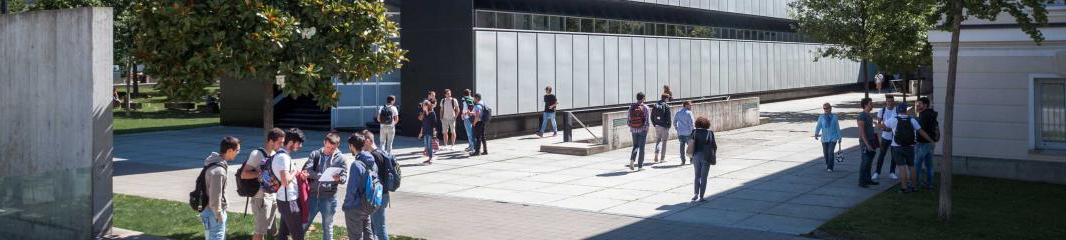USI - Email## On the numerical stability of linear barycentric rational interpolation

#### Host: Igor Pivkin

 Wednesday26.10 USI Campus Est, room D5.01, Sector D // Online on Microsoft Teams12:15 - 14:00
 Chiara FudaPlease click here to join Abstract: The barycentric forms of polynomial and rational interpolation have recently gained popularity, because they can be computed with simple, efficient, and numerically stable algorithms. We show more generally that the evaluation of any function that can be expressed as $r(x)=\sum_{i=0}^n a_i(x) f_i\big/\sum_{j=0}^m b_j(x)$ in terms of data values $f_i$ and some functions $a_i$ and $b_j$ for $i=0,…,n$ and $j=0,…,m$ with a simple algorithm that first sums up the terms in the numerator and the denominator, followed by a final division, is forward and backward stable under certain assumptions. This result includes the two barycentric forms of rational interpolation as special cases. Our analysis further reveals that the stability of the second barycentric form depends on the Lebesgue constant associated with the interpolation nodes, which typically grows with $n$, whereas the stability of the first barycentric form depends on a similar, but different quantity, that can be bounded in terms of the mesh ratio, regardless of $n$. We support our theoretical results with numerical experiments.Biography:Chiara is a third-year PhD student at USI working under the supervision of Kai Hormann in the field of numerical analysis, mainly on barycentric interpolation. She studied Mathematics at the University of Cosenza in both her bachelor and master and she spent 9 months at the University of Valencia during her degree program.